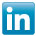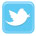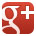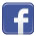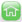» Forum » Inverters » what means of 1:1000 in frequency inverter speed control?
Topics: what means of 1:1000 in frequency inverter speed control? on Inverters
#1

# what means of 1:1000 in frequency inverter speed control?

Hey,

What's the means of 1:1000 in frequency inverter speed control for electric motors?
08-28-2012 05:40 AM
Top #2
This refers to the speed adjusting range of the inverter, specified:

1. Speed adjusting range is the ratio of the frequency inverter at rated load under long-term stable operation of the minimum and maximum speeds, the maximum speed is the rated speed, at this status, this speed range also known as the constant torque speed range. Assuming electric motor is 50Hz / 4 pole motor, no-load speed in theory is: 1500rpm, if speed ratio is 1:1000, the minimum speed is 1.5 rpm. the frequency inverter drives motor in rated torque (load) and stable operation between 1.5-1500 turn

2. For CNC machine, except this indicator, there is another indicator for adjusting speed range, called constant power operating range, the general range is less than 1:5 at the minimum speed rated speed, its maximum speed is equal to K * nominal speed, if it is 1:5, then K = 5, consider the rated 1500rpm, the maximum speed is 7500rpm. Means within the range of 1500-7500rpm, the frequency inverter drives motor constant power operation, due to the speed increases, the torque is getting smaller and smaller.

3. Note: this indicator is reference index for the frequency inverter drives proper motor, electric AC motors are different, the indicators are only useful for reference.
08-28-2012 05:47 AM
Top #3
1:1000 generally refers to the speed range adjustable of the frequency inverter, the lowest the highest. Such as 0.5Hz to 50Hz speed control range 1:100; 0.05Hz to 50Hz speed control range is 1:1000. The inverter can reach the technical indicators, inverter motor is not ancillary to reach.
Share To